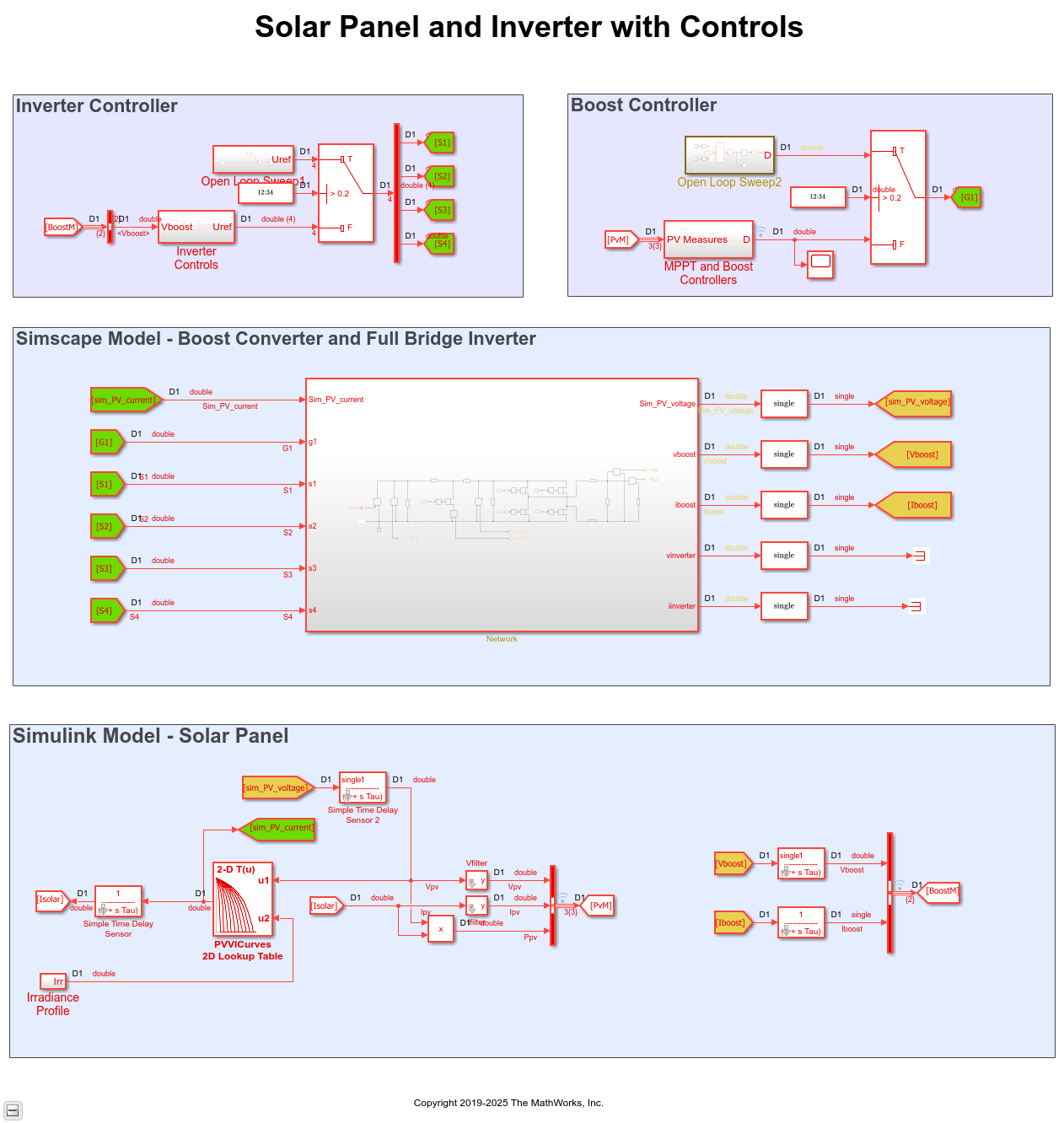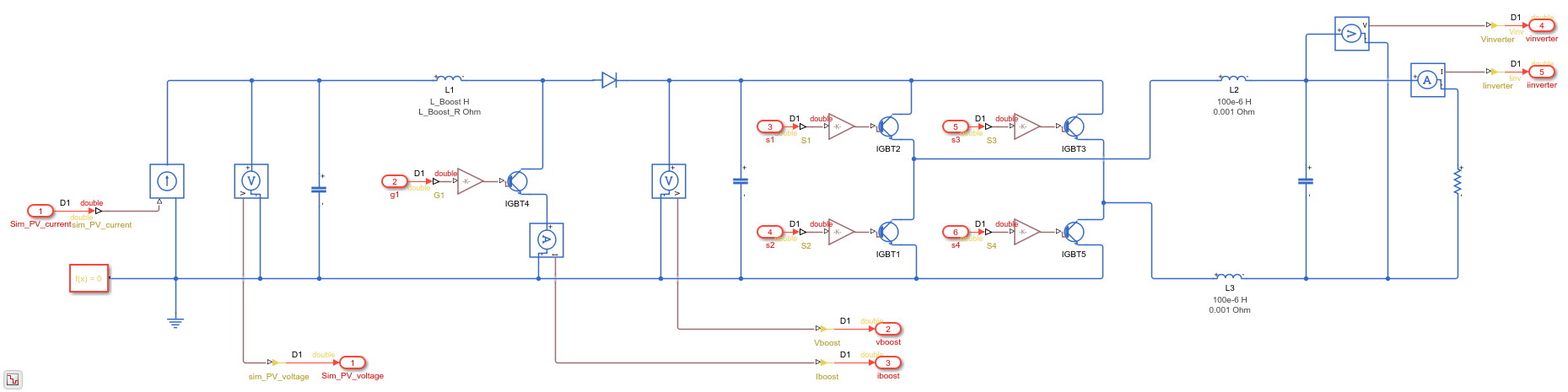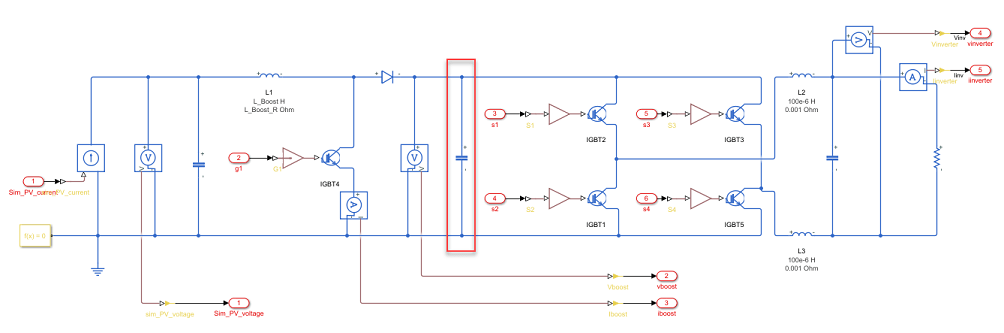# Partition Simscape Models Containing a Large Network into Multiple Smaller Networks

This example shows how to partition a solar power inverter model that contains a single, large Simscape™ network into multiple networks. After you partition the network, you can run the Simscape HDL Workflow Advisor to generate the HDL implementation model. To learn how you run the Advisor for the model, see Generate HDL Code for Simscape Models with Multiple Networks.

### Why Partition a Simscape Network

When your Simscape model contains many switching elements, the state-space representation can contain a large number of modes. The Simscape HDL Workflow Advisor simulates the Simscape model to calculate the number of modes that are relevant. Certain Simscape models can have a large number of modes that are relevant. The generated HDL implementation model for such a large design can consume a significantly large number of resources, and the generated HDL implementation md may even fail to synthesize on the target FPGA device. To reduce the number of modes, you can partition the Simscape network in your model into multiple networks, and then run the Simscape HDL Workflow Advisor.

### Solar Power Inverter Model with Single Network

To open the solar power inverter example model, run:

```open_system('sschdlexSolarInverterSingleNetworkExample') ```

For this example, the model is saved as Solar_Power_Inverter_Single_Network_HDL. This model is the same as sschdlexSolarInverterSingleNetworkExample but has the subsystems rearranged and the logic for the solar panel placed inside a Solar_Panel subsystem.

```open_system('Solar_Power_Inverter_Single_Network_HDL') set_param('Solar_Power_Inverter_Single_Network_HDL', 'SimulationCommand', 'Update') ```The model consists of four parts: solar panel, boost controller, inverter controller, and a boost converter and full bridge inverter. The solar panel is modeled in Simulink® by using lookup tables. The boost controller and inverter controller provide the control signals for the boost converter and the full bridge inverter which is an H-bridge.

To see the boost converter and inverter, open the Network subsystem.

```open_system('Solar_Power_Inverter_Single_Network_HDL/Network') ```### Run Simscape HDL Workflow Advisor

1. To open the Simscape HDL Workflow Advisor for the model, enter:

```sschdladvisor('Solar_Power_Inverter_Single_Network_HDL') ```
```### Running Simscape HDL Workflow Advisor for <a href="matlab:(Solar_Power_Inverter_Single_Network_HDL)">Solar_Power_Inverter_Single_Network_HDL</a> ```

2. Run the workflow to the Discretize Equations task. You see that the state-space representation uses around `173` modes, which is a large number of modes.Such a large number of modes can consume a significantly large number of hardware resources, and may even cause the DUT subsystem in the HDL implementation model to fail to synthesize on the target FPGA device.

### Generate HDL Implementation Model and View Resource Consumption

To see the resource consumption:

1. Run the Generate implementation model task. Click the link to open the HDL implementation model.

The model contains a HDL Subsystem block that models the state-space equations for the Simscape network. Save the model as Solar_Power_Inverter_Single_Network_StateSpace.slx.

```open_system('Solar_Power_Inverter_Single_Network_StateSpace') set_param('Solar_Power_Inverter_Single_Network_StateSpace', 'SimulationCommand', 'Update') ```2. Enable generation of the resource utilization report.

```hdlset_param('Solar_Power_Inverter_Single_Network_StateSpace', 'ResourceReport', 'on') ```

3. Run the `makehdl` function to generate code for the HDL Subsystem block.

```makehdl('Solar_Power_Inverter_Single_Network_StateSpace/HDL Subsystem') ```

If HDL Coder™ generates an error that it is unable to allocate delays, increase the Oversampling factor. Start by increasing the Oversampling factor to `100`, and then generate HDL code. If HDL Coder is still unable to allocate delays, then further increase the Oversampling factor.

```hdlset_param('Solar_Power_Inverter_Single_Network_StateSpace', 'Oversampling', 100) ```

4. As you generate HDL code, open the Code Generation Report. The resource utilization report indicates a large amount of multipliers, adders, and registers that might be consumed on the target FPGA device.### Partition Solar Inverter Network into Multiple Simscape Networks

To reduce the number of modes, you can partition the Simscape network inside the Network subsystem into two Simscape networks. To partition the network into multiple networks:

1. Identify the boundary for partitioning the network into multiple networks. An energy storage element such as a capacitor or an inductor makes a good candidate for partitioning the network. To produce a Simscape model that contains multiple networks and effectively reduces the number of modes in the state-space representation, choose a boundary that produces identical or near identical partitions. That is, the number of switching elements on either side of the boundary are identical or nearly identical.

For the solar power inverter, you can choose the DC link capacitor between the H-bridge inverter and the boost converter as the boundary for partitioning the network.2. After you partition the network, prepare the modified Simscape model for compatibility with the Simscape HDL Workflow Advisor. Place each partitioned network inside a subsystem and use a Solver Configuration block for each network.

The Simscape HDL Workflow Advisor uses the Solver Configuration block to identify each unique network in your Simscape model.

3. For the simulation to converge when using multiple networks, in the network containing the boost converter, add a snubber resistance and a controlled current source in parallel to the capacitor for the current output to the inverter network.4. In the inverter network, add a controlled voltage source to the voltage input to the network.5. To break the algebraic loops in the system, add Delay blocks between the signal lines that connect the output of one subsystem to the input of the other subsystem. For higher accuracy, add Data Type Conversion blocks to provide double data types as inputs to the networks.### Solar Power Inverter Model with Multiple Networks

The single network model is now partitioned into multiple networks. To open the model containing multiple networks, enter:

```open_system('sschdlexSolarInverterPartitionedNetworkExample') ```

To learn how you run the Simscape HDL Workflow Advisor and generate HDL code for this model, see Generate HDL Code for Simscape Models with Multiple Networks.